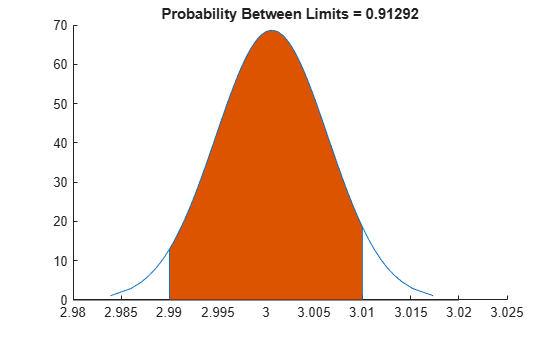Main Content

# capaplot

Process capability plot

## Syntax

```p = capaplot(data,specs) [p,h] = capaplot(data,specs) ```

## Description

`p = capaplot(data,specs)` estimates the mean of and variance for the observations in input vector `data`, and plots the pdf of the resulting T distribution. The observations in `data` are assumed to be normally distributed. The output, `p`, is the probability that a new observation from the estimated distribution will fall within the range specified by the two-element vector `specs`. The portion of the distribution between the lower and upper bounds specified in `specs` is shaded in the plot.

`[p,h] = capaplot(data,specs)` additionally returns handles to the plot elements in `h`.

`capaplot` treats `NaN` values in `data` as missing, and ignores them.

## Examples

collapse all

Randomly generate sample data from a normal process with a mean of 3 and a standard deviation of 0.005.

```rng default; % For reproducibility data = normrnd(3,0.005,100,1);```

Compute capability indices if the process has an upper specification limit of 3.01 and a lower specification limit of 2.99.

`S = capability(data,[2.99 3.01])`
```S = struct with fields: mu: 3.0006 sigma: 0.0058 P: 0.9129 Pl: 0.0339 Pu: 0.0532 Cp: 0.5735 Cpl: 0.6088 Cpu: 0.5382 Cpk: 0.5382 ```

Visualize the specification and process widths.

```capaplot(data,[2.99 3.01]); grid on```## See Also

Introduced before R2006a

Download ebook